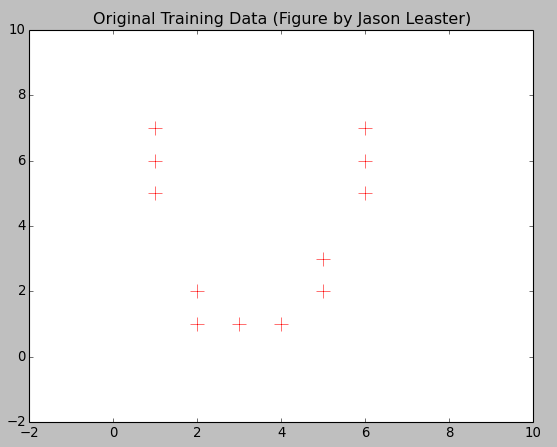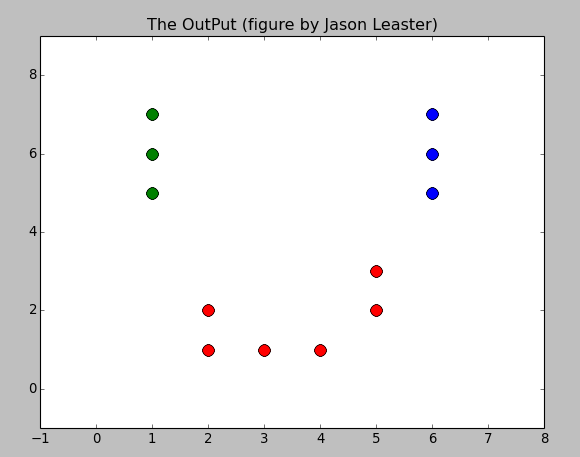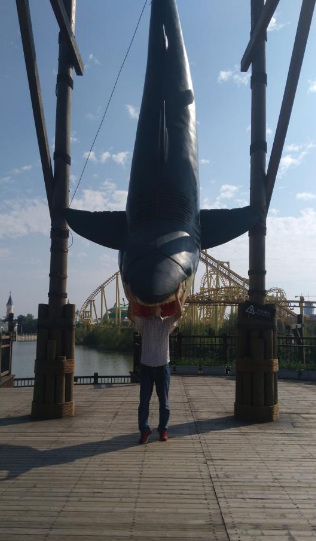K-Means is a classical unsupervised clustering Learning Algorithm. The detail of the theory about K-Means that you can find it in Wikipedia. Now I introduce to implement this algorithm by myself.

If you are interesting in the implementation and change it into a better version, you could find it in my github repository and give me some advices. I will be appreciated.

So consider about if I want to classify the data into three different cluster. How could I make it?Here is the result:With the mean values:

In the implementation, I just choose the euclidean distance equation as my sensor to calculate the distance between samples. You could assign the `self.distance` with your function which is in your application.

Here, I show you how to classify the sample point in `K-Means`.

And, here you will glance at the main procesure of this algorithm.